Question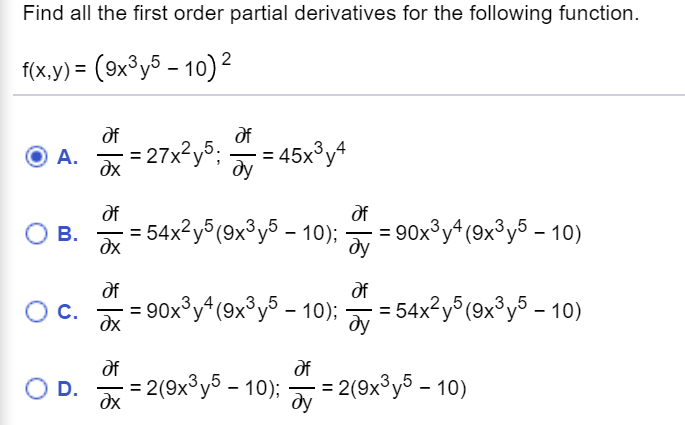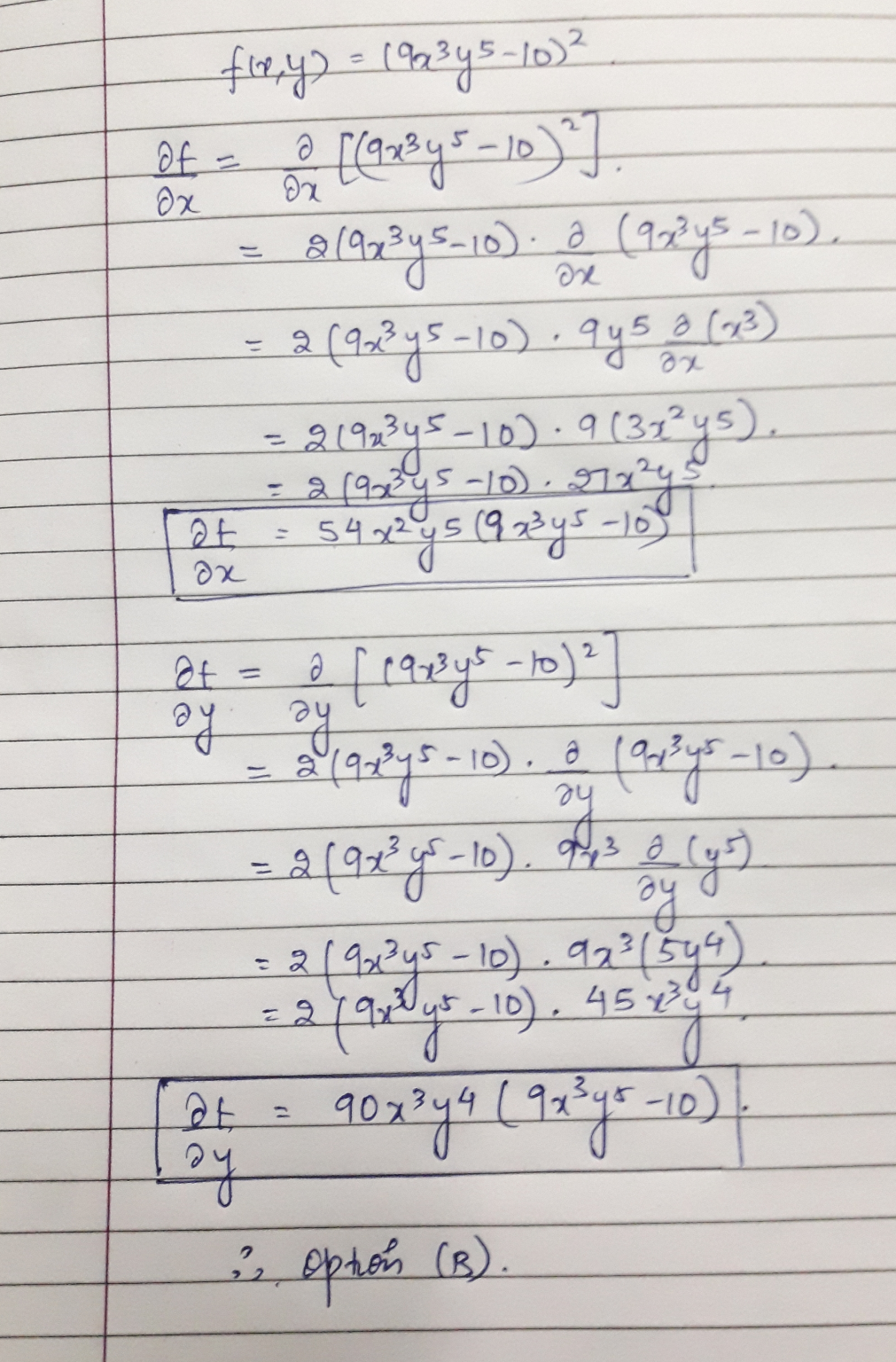#### Earn Coins

Coins can be redeemed for fabulous gifts.

Similar Homework Help Questions
• ### Find the partial derivatives for the following function. Find the partial derivatives for the following function....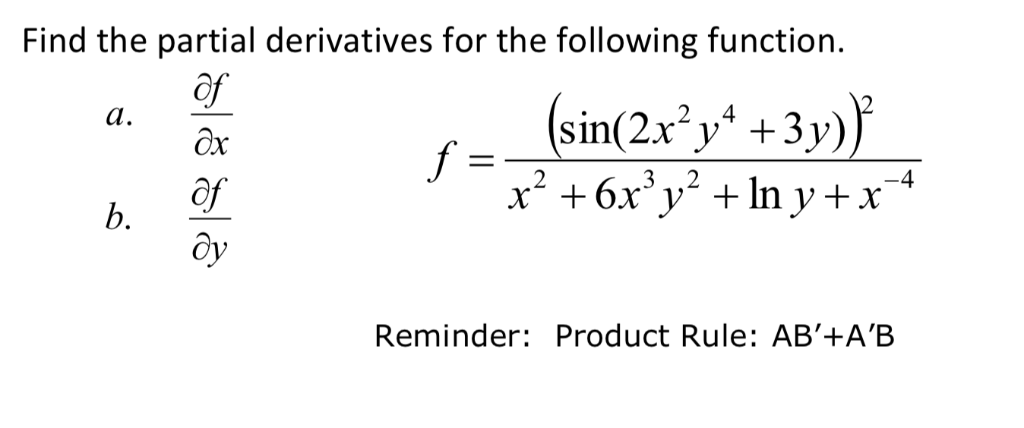Find the partial derivatives for the following function. Find the partial derivatives for the following function. of a. Ox of ду 3 ,,2 b. Reminder: Product Rule: AB'+A'B

• ### Find all the first and second order. partial derivatives of f(x, y) = 8 sin(2x +...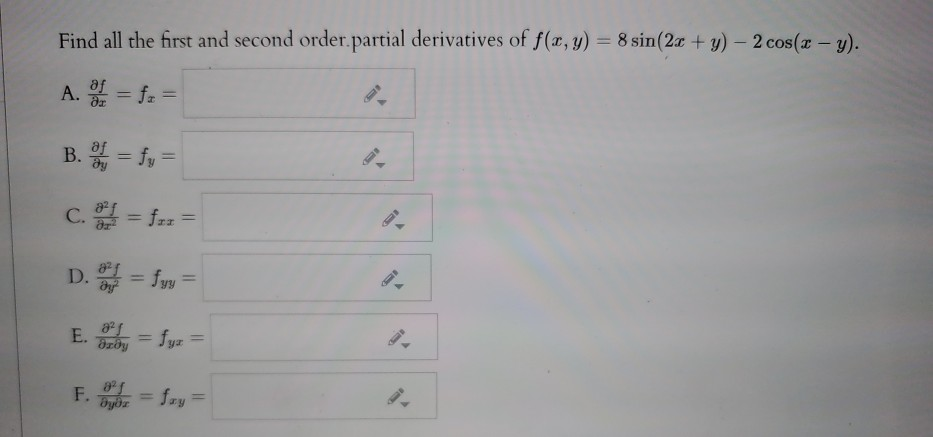Find all the first and second order. partial derivatives of f(x, y) = 8 sin(2x + y) - 2 cos(x - y). A. SI = fr = B. = fy = c. = f-z = D. = fyy = E. By = fyz = F. = Sxy=

• ### Find the first-order partial derivatives (fr. f,) and second-order partial derivatives (fxxıfyy, fxy, fyx) of the...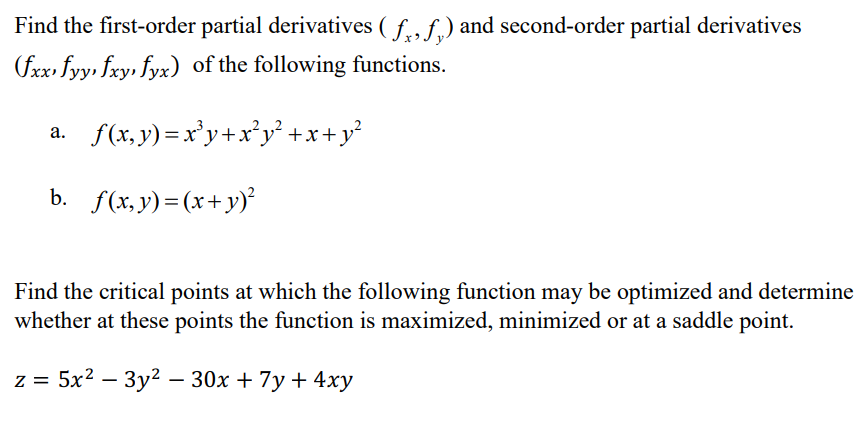Find the first-order partial derivatives (fr. f,) and second-order partial derivatives (fxxıfyy, fxy, fyx) of the following functions. a. f(x,y)=x’y+x’y? +x+y? b. f(x, y) = (x + y)? Find the critical points at which the following function may be optimized and determine whether at these points the function is maximized, minimized or at a saddle point. z = 5x2 – 3y2 – 30x + 7y + 4xy

• ### find all first partial derivatives f(x,y)= 5x^3+4y-3 Find all first partial derivatives. f(x, y) = 5x3...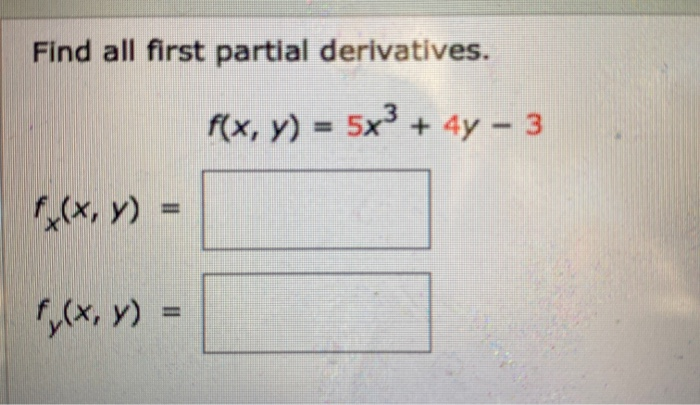find all first partial derivatives f(x,y)= 5x^3+4y-3 Find all first partial derivatives. f(x, y) = 5x3 + 4y - 3 f(x,y) = f(x,y) =

• ### 6 of 15 (complete) Find all the second-order partial derivatives of the function f(x,y) - 7x...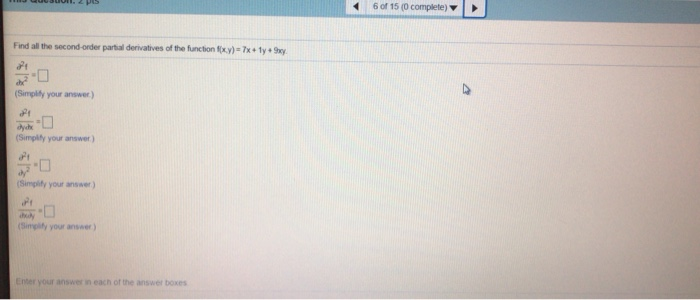6 of 15 (complete) Find all the second-order partial derivatives of the function f(x,y) - 7x + y + xy (Simplify your answer) dyche (Simply your answer) 21 dy (Simplify your answer (Simply your answer Enter your answer in each of the answer boxes

• ### Find all the first order partial derivatives for the following function. - (sin xy)cos yz 2)...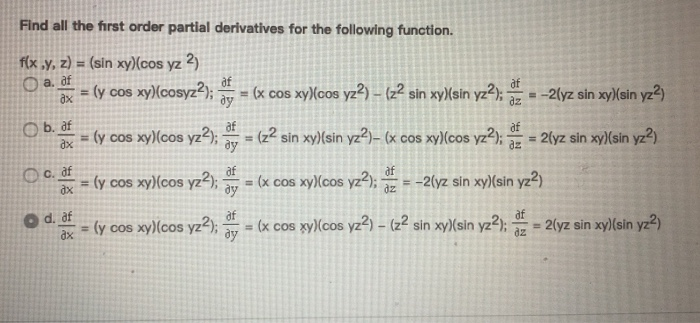Find all the first order partial derivatives for the following function. - (sin xy)cos yz 2) flx ,y, z) y 009 )lcosyain xy)lein ) Co2lyz sin ky/sin 2) df COS Cos 2y2 cos yos v2 - 2lyz in xy)lain y?) ах d. af ах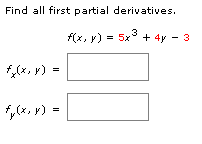Please help me answer these 2 questions Find all first partial derivatives. f(x, y) = 5x + 4y - 3 (, y) = f(x, y) = = Differentiate implicitly to find dy dx xx2 x + y = 5 dy II

• ### 1. Which of the following could represent a function, f (x,y), with first-order partial derivatives ∂ƒ/...

1. Which of the following could represent a function, f (x,y), with first-order partial derivatives ∂ƒ/ ∂x = 3xy (xy + 2) ∂ƒ/ ∂y = x2 (2xy + 3) A. xy (x2y + 3) B. x3y2 + 3x2 – y – 6 C. x2 (xy2 + 3) D. none of these E. x3y2 + 2x2y3 + 1 2. It the consumption function is C = 0.02Y2 + 0.1Y +25. Find the value of Y when MPS = 0.38. 3. State...

• ### Find the all first-order partial derivatives 9. f(x, y, z) = 3x In(x?yz) + xhiz 2...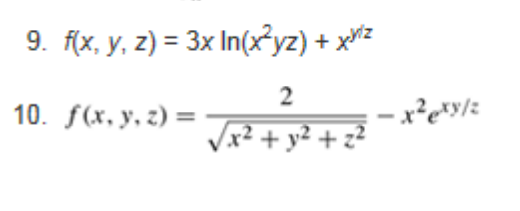Find the all first-order partial derivatives 9. f(x, y, z) = 3x In(x?yz) + xhiz 2 10. f(x, y, z)= 7,21 02 +22 Sin 6. fls. 1) = sin(x – ») + x?tany 7. f(x, y) = ["sindi

• ### Problem 5. (1 point) Find all the first and second order partial derivatives of f(x,y) 7...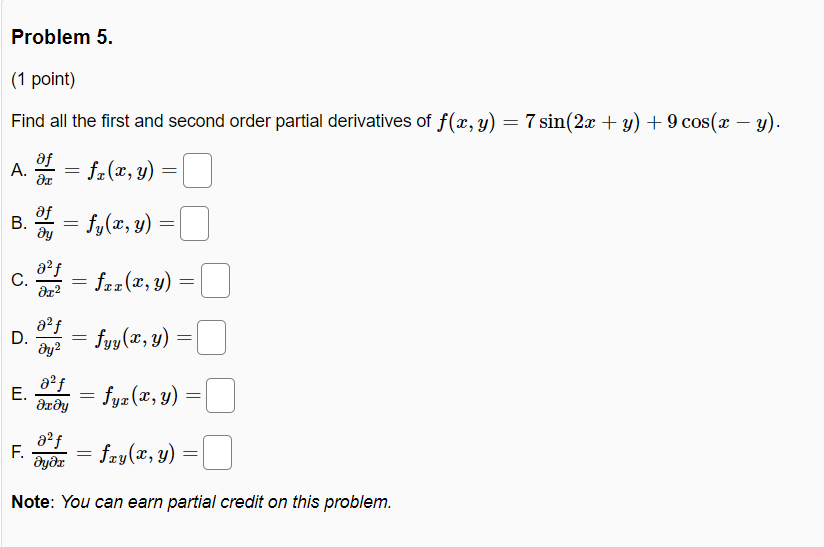Problem 5. (1 point) Find all the first and second order partial derivatives of f(x,y) 7 sin(2x + y) + 9 cos(x - y). A. = fx(x,y) = B. = fy(x, y) = af C. ar2 = fcz(x, y) = af D. ay2 = fyy(x,y) = E. af деду fyz(x, y) = af F. მყმz = fxy(x, y) = Note: You can earn partial credit on this problem.